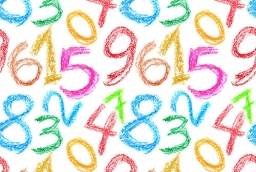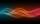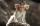# Two numbers 6

Fill two natural numbers a, b:

7 + blank- blank = 5

Correct result:

a =  6
b =  8

#### Solution:Our examples were largely sent or created by pupils and students themselves. Therefore, we would be pleased if you could send us any errors you found, spelling mistakes, or rephasing the example. Thank you!

Please write to us with your comment on the math problem or ask something. Thank you for helping each other - students, teachers, parents, and problem authors.

Showing 1 comment:Dr Math
a = any whole number
b = a+2

i.e.

a = 6
b = 8Tips to related online calculators
Do you have a linear equation or system of equations and looking for its solution? Or do you have quadratic equation?

## Next similar math problems:

• Candy and boxesWe have some number of candy and empty boxes. When we put candies in boxes of ten, there will be 2 candies and 8 empty boxes left, when of eight, there will be 6 candies and 3 boxes left. How many candy and empty boxes left when we put candies in boxes of
• ModuloFind x in modulo equation: 47x = 4 (mod 9) Hint - read as: what number 47x divided by 9 (modulo 9) give remainder 4 .
• LineStraight line passing through points A [-3; 22] and B [33; -2]. Determine the total number of points of the line which both coordinates are positive integers.
• Positive integersSeveral positive integers are written on the paper. Michaella only remembered that each number was half the sum of all the other numbers. How many numbers could be written on paper?
• MistakeNicol mistake when calculate in school. Instead of add number 20 subtract it. What is the difference between the result and the right result?
• IntegerFind the integer whose distance on the numerical axis from number 1 is two times smaller as the distance from number 6.
• Job applicantsJob applicants: three-fourths of applicants had experience for a position. The number that did not have prior experience was 36. How many people applied for the job?
• Mysterious numberThe magician thinks the number: "The mysterious number is first divided by minus five, dividing the result by three, multiplying the number by ten, and dividing the resulting number by minus four. This gives result 5. Can you reveal the mysterious number
• 15 numberWhat number is smaller (greater) by 15 than its half?
• Unknown xIf we add to unknown number 21, then divide by 6 and then subtract 51, we get back an unknown number. What is this unknown number?
• SequenceIn the arithmetic sequence is a1=-1, d=4. Which member is equal to the number 203?
• Four integersFnd four consecutive integers so that the product of the first two is 70 times smaller than the product of the next two.
• CZK coinsThaddeus and Jolana together have 15 CZK. Jolana has half of Thaddeus money. Nevertheless Jolana has 3 coins and Thaddeus 2 coins. Which coin has Thaddeus and Jolana (Help: CZK coins has values 1,2,5,10,20,50 CZK)?
• RepairmanRepairman has vowed to do repair work at the plant for 25 days. However work had to be shortened, and therefore he took helper worker. Together they made all the corrections for the whole days. How long it would take work to helper worker?
• SimplifySimplify expression - which expression is equivalent to: 3(m + 2) − 4(2m − 9)
• EquatiomSolve equation with negatives: X/(-5) + 2 = -9
• Balance of accountTheo had a balance of -$4 in his savings account. After making a deposit, he has$25 in his account. What is the overall change to his account?